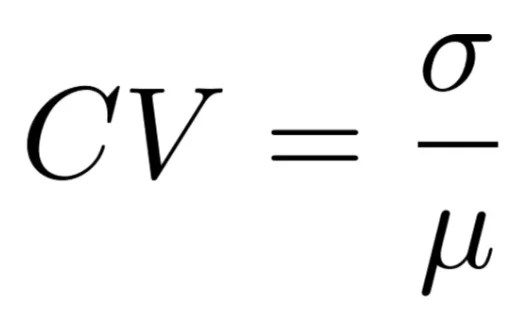# Co-efficient of Variation

8th February 2020

The coefficient of variation (CV) is a statistical measure of the dispersion of data points in a data series around the mean. The coefficient of variation represents the ratio of the standard deviation to the mean, and it is a useful statistic for comparing the degree of variation from one data series to another, even if the means are drastically different from one another.

#### The Formula for Coefficient of Variation isWhere: σ is the standard deviation and μ is the mean.

The coefficient of variation shows the extent of variability of data in sample in relation to the mean of the population. In finance, the coefficient of variation allows investors to determine how much volatility, or risk, is assumed in comparison to the amount of return expected from investments. The lower the ratio of standard deviation to mean return, the better risk-return trade-off. Note that if the expected return in the denominator is negative or zero, the coefficient of variation could be misleading.

The coefficient of variation is helpful when using the risk/reward ratio to select investments. For example, an investor who is risk-averse may want to consider assets with a historically low degree of volatility and a high degree of return, in relation to the overall market or its industry. Conversely, risk-seeking investors may look to invest in assets with a historically high degree of volatility.

While most often used to analyze dispersion around the mean, quartile, quintile, or decile CVs can also be used to understand variation around the median or 10th percentile, for example.

• The coefficient of variation (CV) is a statistical measure of the dispersion of data points in a data series around the mean.
• In finance, the coefficient of variation allows investors to determine how much volatility, or risk, is assumed in comparison to the amount of return expected from investments.
• The lower the ratio of standard deviation to mean return, the better risk-return trade-off.